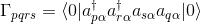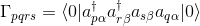# Extracting alpha and beta densities

Dear All,

Is there a way to extract the alpha and beta densities from a full CI calculation? The program clearly builds the densities separately and diagonalizes them to provide the alpha and beat Natural occupations. Is there a way to get the Natural orbitals of each density as well - maybe to a molden file.

Best,
Shachar

Probably the best way is to grab the density from the CIWavefunction:

set opdm True
ci_energy, ci_wfn = energy('FCI', return_wfn=True)
opdm_a = ci_wfn.get_opdm(1, 1, "A", True)


The above will return the alpha OPDM between roots 1 and 1, this routine can return any OPDM built including transition densities. “A” can be replaced by “SUM”/“B” for the total/beta densities and the final True means to return the OPDM in the full space (if False only the active space). These densities are all in the MO basis.

The Molden writer is not setup to handle this kind of operation natively. It is possible to build a script to do so however.

Dear Daniel,

Thanks for answering. I am a bit new to the program and I can’t seem to make your suggestion work.

When I run the “ci_energy, ci_wfn = energy(‘FCI’, return_wfn=True)” the program runs the same but I get the following error:

Traceback (most recent call last):
File “”, line 27, in
TypeError: ‘float’ object is not iterable

Assuming this can be easily overcome, does opdm_a in your suggestion contain the density matrix in MOs or already the NOs and in which basis? How can I access the data, i.e., is it a normal array ?

I tried several option, e.g., print opdm_a, opdm_a.print_out() , but got nothing out.

Thanks again.

Best,
Shachar

Im guessing you have an older version of Psi4 before the Wavefunction passing update. Please update to the latest Psi4 version for this to work.

opdm_a would be the <1|E_{tu}|1> OPDM in the MO basis. If it was in the NO basis it would simply be diagonal. This is a normal Psi4 matrix which you can access as you have shown. See the below example for a NumPy version:

import numpy as np

molecule mol {
He
symmetry c1
}

set basis cc-pvdz
set opdm True
ci_energy, ci_wfn = energy('FCI', return_wfn=True)

matrix_opdm_a = ci_wfn.get_opdm(0, 0, "A", True)

# Prints to psi4 output
matrix_opdm_a.print_out()

# Only works in C1 symmetry and prints to screen below this line
opdm_a = np.array(matrix_opdm_a)

# Diagonalize the OPDM
eigvals, eigvecs = np.linalg.eigh(opdm_a)

# Numpy likes to return them backwards, need to reverse them!
eigvals = eigvals[::-1]
eigvecs = eigvecs[:, ::-1]

# Natural orbital occupation numbers
print 'Occupation numbers:'
print eigvals

# Rotate current orbitals to natural orbitals
nat_orbs_a = np.dot(ci_wfn.Ca(), eigvecs)
print 'Natural orbitals:'
print nat_orbs_a

I think it would be really useful to have snippets like these saved for future reference in some central place.
Even a gist link in the manual would do. Just a thought.

Good idea, @hokru. The trick will be to keep them working. It’s a little too involved to write compare-against-stored-value test cases for snippets. But we may be able to include them as tests so that if they throw an error the test case fails, so we notice and fix the error or update the snippet. All tests get installed to share/psi4/samples

Dear Daniel,

Thank you very much for the script. It’s really wonderful that one can access the information so easily.

If I would like to implement it for higher symmetry I would also need the SALC matrix to transform back to the basis set AOs. Is it also available under wfn ?

In hopes of becoming more self reliant, is there somewhere which lists all the accessible function from a given class, e.g., wfn.Ca, wfn.get_opdm etc. and what they return ?

Thank you again.

Best,
Shachar

It hasn’t had its docstrings filled out as much as they ought to be, but all the classes/fns/etc. are autodoc-ed here.

@shachar Ill be out of the office for the next few days so my replies will be spotty. Probably the easiest answer is to get NumPy arrays for each irrep of the OPDM:

list_of_arrays = [np.array(x) for x in ciwfn.get_opdm(0, 0, "A", True).array_interfaces()]


I have been thinking about this a bit more and it would be nice if the Molden writer would natively support these kind of operations. I will generalize the Molden function to carry out these operations when I get back.

You can also check out specifically the CIWavefunction class that is returned.

A lot of Psi4’s low level infrastructure is available at the python level and we do not do a great job of documenting it yet. This is on a summer TODO list, so hopefully this will become a more standard feature.

Thanks for all the advice. I currently manages to write the densities into a molden file using the following script:

    ci_energy, ci_wfn = energy('FCI', return_wfn=True)
nmo=ci_wfn.nmo()
nso=ci_wfn.nso()
# Alpha Density
CA = ci_wfn.Ca()
DA = ci_wfn.Da()
NOSA = psi4.Matrix(nmo,nmo);
NOSOA = psi4.Matrix(nso,nmo);
NOOCA = psi4.Vector(nmo);
DA.diagonalize(NOSA,NOOCA,DiagonalizeOrder.Descending)
NOSOA.gemm(False, False, 1.0, CA, NOSA, 0.0)
# Beta Density
CB = ci_wfn.Cb()
DB = ci_wfn.Db()
NOSB = psi4.Matrix(nmo,nmo);
NOSOB = psi4.Matrix(nso,nmo);
NOOCB = psi4.Vector(nmo);
DB.diagonalize(NOSB,NOOCB,DiagonalizeOrder.Descending)
NOSOB.gemm(False, False, 1.0, CB, NOSB, 0.0)
# Total Density
D = psi4.Matrix(nmo,nmo);
D.zero()
NOS = psi4.Matrix(nmo,nmo);
NOSO = psi4.Matrix(nso,nmo);
NOOC = psi4.Vector(nmo);
D.diagonalize(NOS,NOOC,DiagonalizeOrder.Descending)
NOSO.gemm(False, False, 1.0, CA, NOS, 0.0)
# Molden File
mw = psi4.MoldenWriter(ci_wfn)
mw.write('AB.molden', NOSOA, NOSOB, NOOCA, NOOCB, NOOCA, NOOCB)


The molden write is a bit annoying in the sense that it orders the nos in an ascending manner. Nevertheless, it works. There is a writeNO function for MoldenWrite but this is not enabled for the python interface. This might be a very simple change and would save much of the script. I am trying to generalize this for general symmetry but I am not there yet. This might also be solved if writeNO would be enabled since it will take the psi.Matrix in the MO basis directly.

Since I am still rather new to all these environment variables I would appreciate any comments anyone might have on the script.

Best,
Shachar

@Shachar This looks great! Pretty close to what I am going to do in the Molden wrapper. A few points:

• You should be able to replace nmo with nmopi() and nso with nsopi() to enable the usage of symmetry. nmopi() is number of molecule orbitals per irrep which effectively returns a small list.
• The Da and Db slots in the wavefunction should always be in the SO basis. I did not do this originally for the CI code and this will be fixed very soon which will break the above code. You can either transform into the MO yourself or use the get_opdm function which will always be density matrices in the MO basis.
• You can always add writeNO to the Psi4 python driver yourself!

If you are unfamiliar with git pull request model there is a short Psi4 tutorial here.

Add the following writeNO line to src/bin/psi4/export_mints.cc:954:

class_<MoldenWriter, boost::shared_ptr<MoldenWriter> >("MoldenWriter", "docstring", no_init).
def(init<boost::shared_ptr<Wavefunction> >()).
def("writeNO", &MoldenWriter::writeNO, "docstring"),    //add this line!
def("write", &MoldenWriter::write, "docstring");


Ill probably get around to doing this in a few days, but feedback on the push/pull process is always greatly appreciated!

There seems to be a problem with the above code in the case where one of the irreps is “empty”. I also looked at the SOTOAO.dat code you have in the PSI4NUMPY project and the same problem occurs there so I guess this the result of some more recent change.

I guess I could check if ‘x’ is empty before applying np.array which results in an exception.TypeError, but I was wondering if I might be missing something.

Best,
Shachar

@shachar NumPy does have issues handling Psi arrays that have no elements. This is still an open area of discussion. Perhaps a better solution right now is to use:

list_of_arrays = ciwfn.get_opdm(0, 0, "A", True).to_array()


This will return a list of NumPy arrays where Psi irreps without elements will be np.empty objects. The alternative is to do the following:

list_of_arrays = ciwfn.get_opdm(0, 0, "A", True).to_array(dense=True)


which will expand the Psi irreped matrix into a simple dense NumPy matrix. This has not been exported to the docs yet, but the file that does this along with documentation is located at psi4/share/python/p4util/numpy_helper.py.

On a related note, can anyone clarify the ordering of the two-particle density matrix that is exported by similar commands:

tpdm_array_aa = np.array(cisd_wfn.get_tpdm("AA",False))
tpdm_array_ab = np.array(cisd_wfn.get_tpdm("AB",False))
tpdm_array_bb = np.array(cisd_wfn.get_tpdm("BB",False))


My intuition would be a matricized 4-index tensor given by a M^2 by M^2 matrix for each of the TPDMs indexed by spatial orbital. There are some convention issues often associated with TPDMs however. For example some write the TPDM as
D[ij,kl] = <| a_i^\dagger a_j^\dagger a_l a_k |> (note the transposed l and k, which introduces a - sign). However, ignoring for now the (-) sign, it’s not totally obvious to me from the source code the ordering that is used in the “AB” tensor. That is, is it
D[ij,kl] = <| a_{i \alpha}^\dagger a_{j \beta}^\dagger a_{l \alpha} a_{k \beta} |>
or perhaps
D[ij,kl] = <| a_{i \alpha}^\dagger a_{j \alpha} a_{k \beta}^\dagger a_{l \beta} |>
which wouldn’t make a ton of sense to me given typical definitions, but I can’t find any reference for the internal ordering used, so I have to assume almost anything is possible! Any help in this regard would be appreciated.

Good point, ill add these notes to the code. Should follow the basic work of Olsen and Co. The AA term is:The AB term is:Id like to point out that this sort of functionality is really in beta and the CIWavefunction will be changing soon. If you are using this functionality for extremely low-level work please contact me so that I do not write out the sections that you may need.